# How to choose a trend line

In a scatter plot , a trend line is great for showing a correlation in your data. If your data points are scattered all over the chart, a trend line is useless – but if you see a trend in the data that you want to show, Datawrapper can draw a trend line for you.

## How to add a trend line

You can find the option Trend line at the bottom of Refine tab in Step 3: Visualize: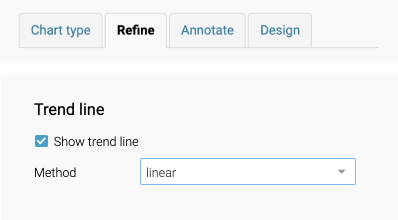You have 7 options: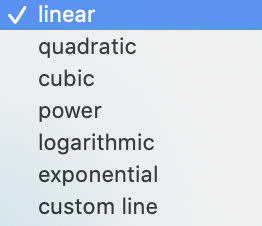## Which trend line to choose

#### Should you draw a trend line at all?

First, it may be a good idea to question whether it's worth drawing a trend line at all. If the data is forced to fit a trend line, it may be more misleading than helpful.

#### Choose the line that fits the data best

Ideally, you should choose the trendline where all data points have the smallest distance to the trendline.

That is, in the image below, to keep the total distance of the red lines as small as possible: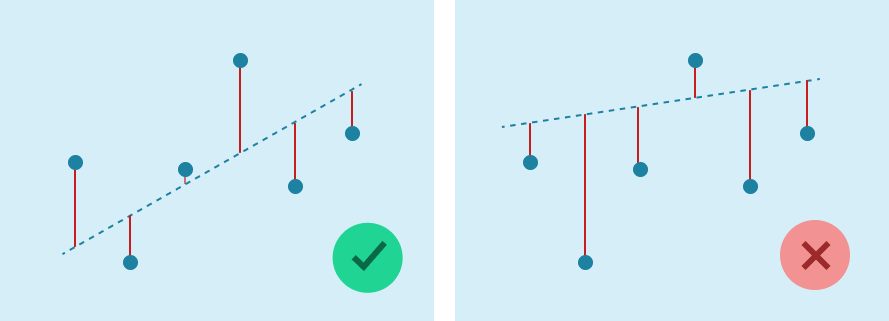(This accuracy is sometimes shown as the R-squared value or the coefficient of determination (a number from 0 to 1 where 1 is the most accurate). Datawrapper doesn't calculate this for you, but calculating it might help you determine whether a trend line is a good enough fit or not.)

## Trend lines you can draw in Datawrapper

There are many ways you can draw a trend line. Datawrapper allows you to draw some of the most useful ones:

### Linear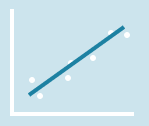If your data values increase/decrease at a constant rate and resemble a straight line, then choose linear. This is probably the most common trend line and the one that's easiest to understand.

### Custom

If your data values resemble a straight line but selecting linear doesn’t fit the data best, then you can also draw one yourself by selecting custom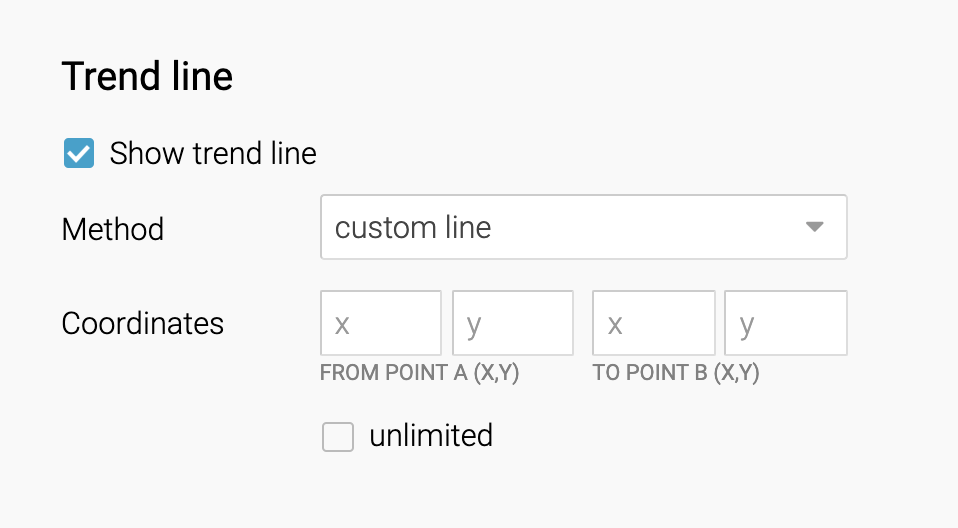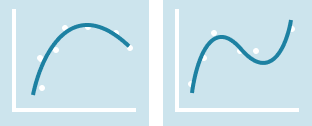If your data fluctuates (goes up and down) and resembles a curved line, choose quadratic or cubic. These are part of what's called the polynomial trend linesQuadratic (Order 2 polynomial trend line) has one curve in the trend line. Cubic (Order 3) has two curves.

### Exponential, Logarithmic, Power

If your data values don't resemble a straight line or a fluctuating curve but increase or decrease rapidly then consider either exponential, logarithmic, or power.

#### Exponential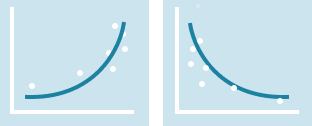If your data increases at an increasing rate or decreases at a decreasing rate, then exponential might be a good fit. The exponential curve cannot take zeros or negative values.

#### Logarithmic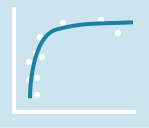If your data increases rapidly but then flattens to a plateau, logarithmic might be a good fit.

#### How the trend lines look differently when choosing a log scale

If your data plot looks exponential and logarithmic, you might also want to consider changing one of your axes to a log scale.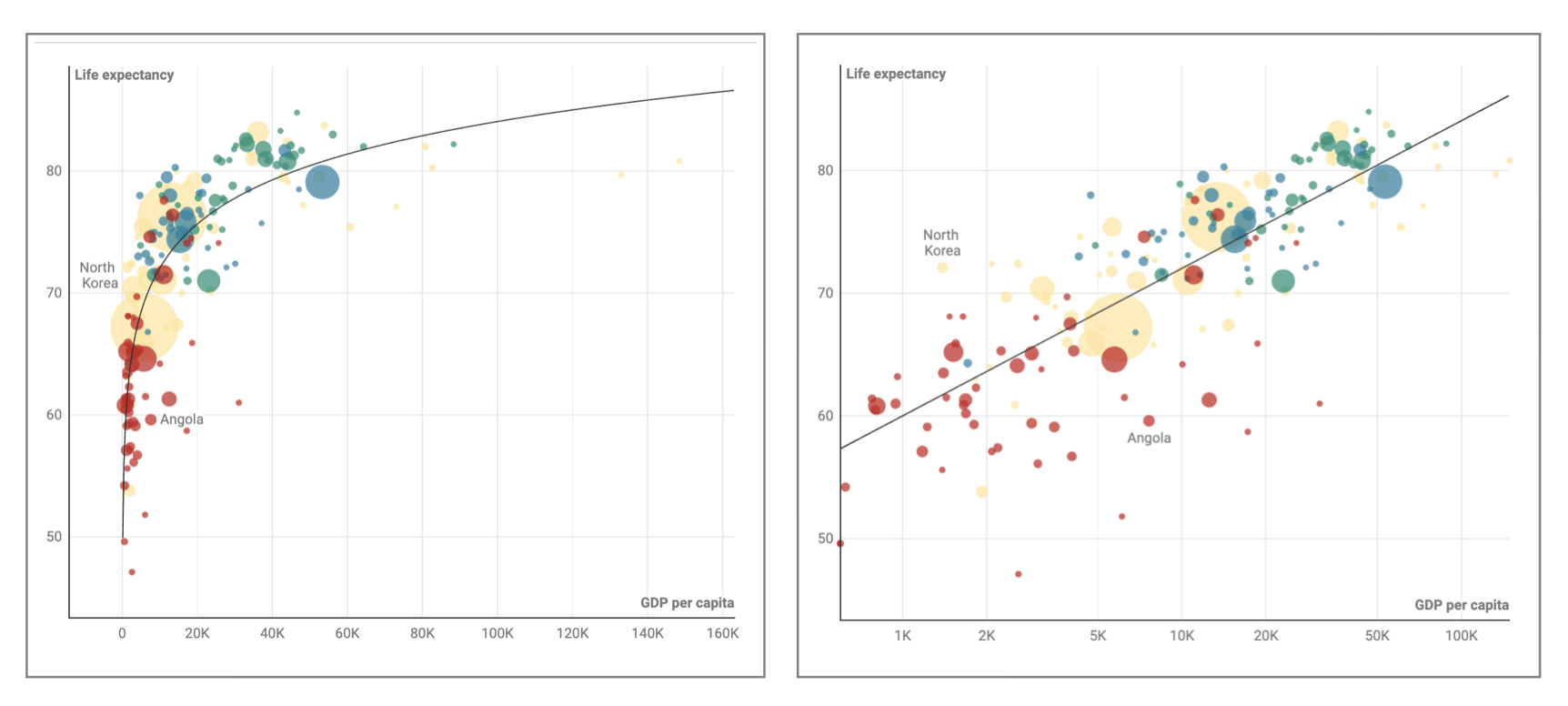You can learn more about log scales in our 3-part blog post about log scale. You can either turn one axis into a log scale or both axes into log scales and selecting the following options will draw a straight-line correlation between the two variables.

• Linear-log plot (when the vertical axis is a log scale): choose exponential - this type of trend is common in data with exponential growth (e.g. COVID case numbers) or exponential decay (e.g. decaying time of radioactive substance)
• Log-linear plot (when the horizontal axis is a log scale): choose logarithmic - this is less common compared to linear-log plot but for example used to show when data on the horizontal axis is unevenly distributed toward one end of the scale (e.g. Big Bang timeline, frequency distribution, pH distribution, etc.)
• Log-log plot (when both axes are in log scales): choose power - when both axes are log scales, choosing a power trend line will draw a straight trend line between the two variables.

These are just some general hints and there are many other ways to calculate and determine the best-fit trend line for your data.

If you want to know how the trend lines are calculated in Datawrapper, they are based on the Javascript module regression-js so take a look at their GitHub page for details.

If you have any questions, reach out to us at support@datawrapper.de